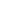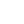# 2019 Craft, Food & Wine Show

• Share:
•
Name: 2019 Craft, Food & Wine Show
Date: November 1, 2019 - November 2, 2019
Event Description:The annual Craft, Food and Wine Show hosted by the St. Lawrence County Chamber of Commerce will be showcasing local and regional artisans, producers, wineries, and crafters.
120+ vendors in all! Local & regional wines and distilled liqueurs, food, jewelry, quilts, furniture, photography, artwork and much more! **New this year - Parking next to Maxcy Hall!

Please see vendor listing below. (updated daily)Click on image to printClick on image to print

Thank you to our sponsors Community Bank, N.A. and <span blockkey="2dv0b" class="_247o" contentstate="c { " entitymap":="" [object="" object],="" "blockmap":="" orderedmap="" {="" "doodn":="" c="" "key":="" "doodn",="" "type":="" "unstyled",="" "text":="" "the="" annual="" craft,="" food="" and="" wine="" show="" hosted="" by="" the="" st.="" lawrence="" county="" chamber="" of="" commerce="" will="" be="" showcasing="" local="" regional="" artisans,="" producers,="" wineries,="" crafters.="" you'll="" find="" beer,="" food,="" jewelry,="" quilts,="" furniture,="" photography,="" artwork="" much="" more!="" **new="" this="" year="" -="" atm="" onsite="" parking="" next="" to="" maxcy="" hall!",="" "characterlist":="" list="" [="" b="" "style":="" orderedset="" {},="" "entity":="" null="" },="" }="" ],="" "depth":="" 0,="" "data":="" map="" {}="" "4p0k":="" "4p0k",="" "",="" [],="" "3oavj":="" "3oavj",="" "for="" a="" complete="" vendors="" more="" info,="" visit:="" http:="" bit.ly="" craftfoodwineshow2019",="" "cbqgl":="" "cbqgl",="" "eprti":="" "eprti",="" "save="" dates!",="" "a6fap":="" "a6fap",="" "rvgq":="" "rvgq",="" "friday,="" november="" 1st="" 12="" 8pm="" suny="" potsdam's="" hall="" ",="" "23t0n":="" "23t0n",="" "saturday,="" 2nd="" 9am="" 4pm="" "ba559":="" "ba559",="" "bc7uc":="" "bc7uc",="" "\$5="" entrance="" fee="" (kids="" under="" are="" free)",="" "1t2n0":="" "1t2n0",="" "a="" free="" shuttle="" available="" from="" other="" potsdam="" lots="" for="" shopper="" convenience.="" "ad72k":="" "ad72k",="" "2dv0b":="" "2dv0b",="" "thank="" you="" our="" sponsors="" arc="" jefferson="" lawrence,="" community="" bank,="" n.a.="" united="" helpers.",="" "6"="" "24"="" "65"="" "2lnrs":="" "2lnrs",="" information="" please="" contact="" commerce,="" 315="" 386-4000.",="" "selectionbefore":="" "anchorkey":="" "anchoroffset":="" 100,="" "focuskey":="" "focusoffset":="" "isbackward":="" false,="" "hasfocus":="" true="" "selectionafter":="" }"="" data-offset-key="2dv0b-5-0" decoratedtext="United Helpers" end="99" entitykey="65" offsetkey="2dv0b-5-0" spellcheck="false" start="85" style="background-color: rgb(220, 230, 248); font-family: Helvetica, Arial, sans-serif; color: rgb(28, 30, 33); white-space: pre-wrap;">United Helpers
.
Location:
SUNY Potsdam
Maxcy Hall Fieldhouse
5 Tupper Lake Drive
Potsdam, NY 13676
Date/Time Information:
Show Hours

Friday, Nov. 1st, 12 pm - 8 pm
Saturday, Nov. 2nd, 9 am - 4 pm

Contact Information:
Jo Ann Roberts
Kids 12 and under are free
Set a Reminder:Places to StayShoppingDiningOutdoor RecreationArt, Culture & HeritageGetting HereCommunity CalendarRequest Info